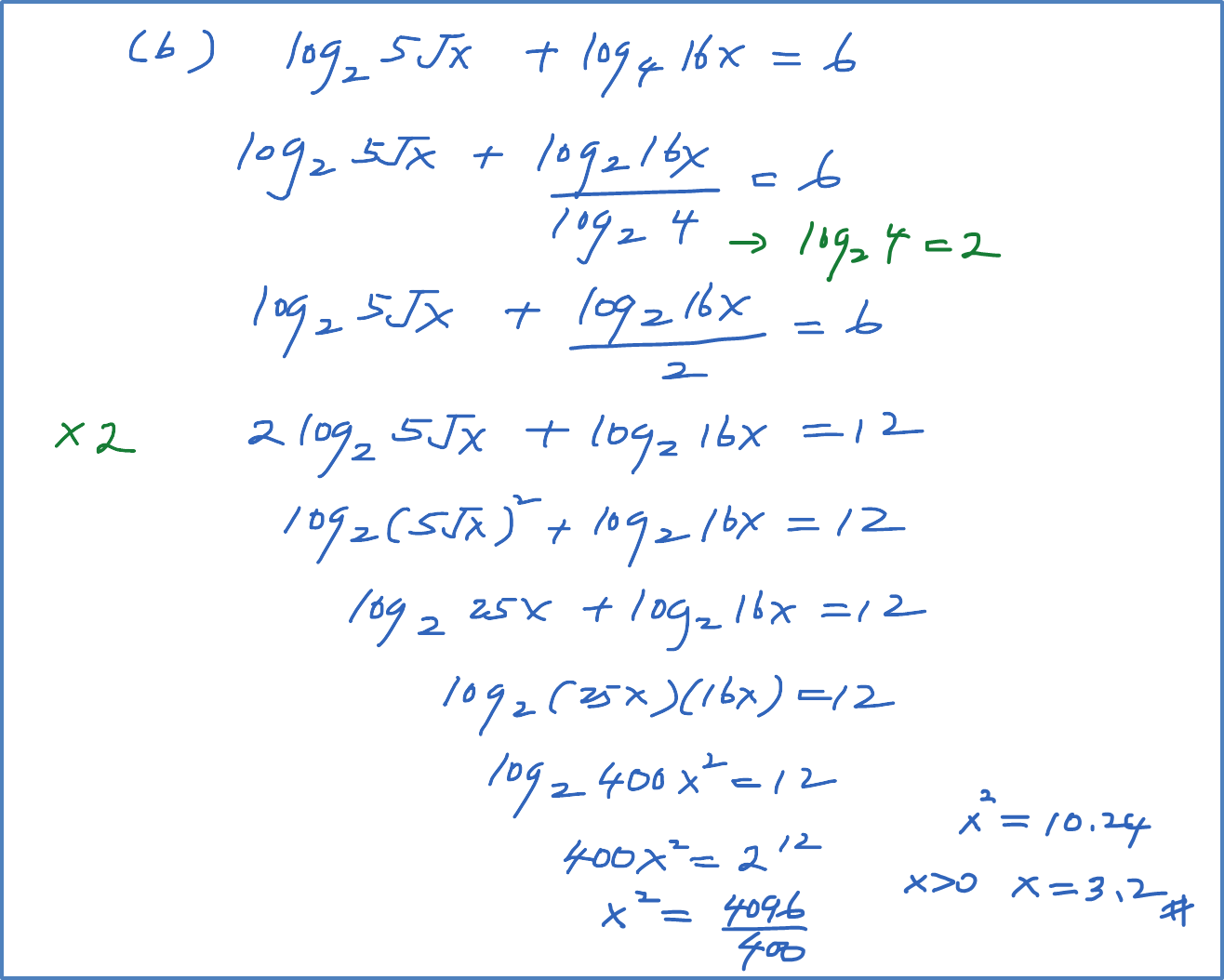# 4.4.5 Logarithms Equation – Example 5

Example 5
Solve the following :
(a) ${\mathrm{log}}_{4}x=25{\mathrm{log}}_{x}4$
(b) ${\mathrm{log}}_{2}5\sqrt{x}+{\mathrm{log}}_{4}16x=6$